### Home > CALC > Chapter 2 > Lesson 2.2.3 > Problem2-81

2-81.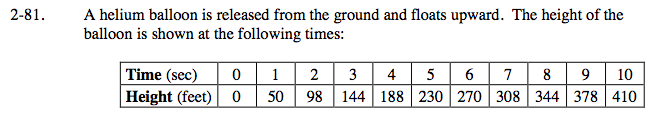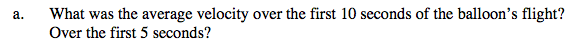In the case of the helium balloon.

$\text{average velocity }=\frac{\Delta\text{height}}{\Delta\text{time}}$

average velocity between t = 0 and t = 10:

$\frac{h(10)-h(0)}{10-0}=\frac{410-0}{10-0}=41 \text{ft/sec}$

Now find the average velocity between t = 0 and t = 5.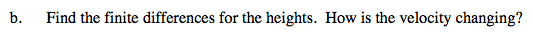This is an example of a finite difference table.

Use the eTool below to explore this.
Click on the link below to view the full version of the eTool. Calc 2-81 HW eTool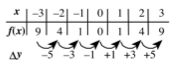In this story, finite differences (Δy) represent the average velocity over consecutive one second intervals.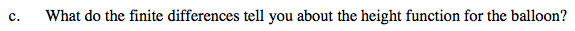Is the height of the balloon increasing, decreasing or both? How do finite differences help you answer this?